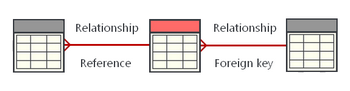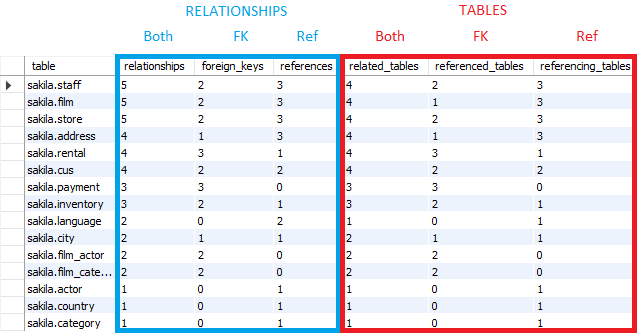## List tables with most relationships in MariaDB database

Article for:

The query below lists tables with most relationships (both foreign keys and FK references from other tables).## Query

``````select concat(database_name, '.', table_name) as 'table',
count(referenced_table) + count(referencing_table) as relationships,
count(referenced_table) as foreign_keys,
count(referencing_table) as 'references',
count(distinct related_table) as related_tables,
count(distinct referenced_table) as referenced_tables,
count(distinct referencing_table) as referencing_tables
from (
(select constraint_schema
as database_name,
table_name
as table_name,
concat(unique_constraint_schema, '.', referenced_table_name)
as referenced_table,
null as referencing_table,
concat(unique_constraint_schema, '.', referenced_table_name)
as related_table
from information_schema.referential_constraints)
union all
(select unique_constraint_schema as database_name,
referenced_table_name as table_name,
null as referenced_table,
concat(constraint_schema, '.', table_name)
as referencing_table,
concat(constraint_schema, '.', table_name)
as related_table
from information_schema.referential_constraints)
) x
group by database_name,
table_name
order by relationships desc;
``````

## Columns

• table - name of the table
• relationships - number of relationships (FKs and FK references)
• foreign_keys - number of foreign keys in a table
• references - number of FK references from other tables
• related_tables - number of different related tables (regardless of relationship type/direction table is counted only once)
• referenced_tables - number of different tables referenced with FKs (please note that table can be related more than once so number of FKs and number of referenced tables can be different)
• referencing_tables - number of different tables referencing with foreign keys this table

## Rows

• One row: represents one table in a database (schema)
• Scope of rows: all tables in a database (schema)
• Ordered by: number of relationships (foreign keys and references) showing the ones with the most first

## Sample results

Sample results in the Sakila database (schema)0